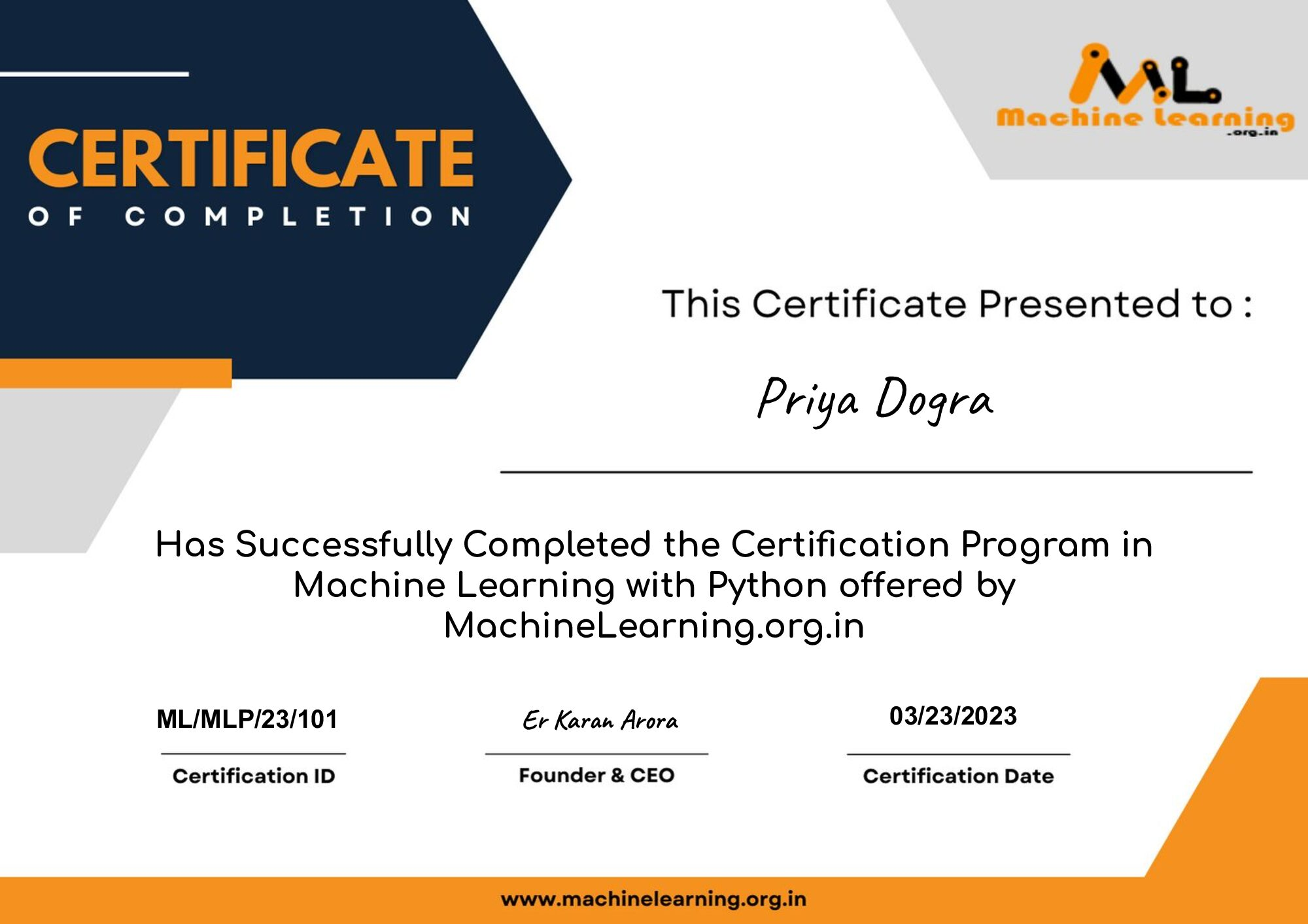Home Quiz Machine Learning with Python Free Certificate

# Machine Learning with Python Free Certificate

0Get Machine Learning with Python Certificate from MachineLearning.org.in which you can share in the Certifications section of your LinkedIn profile, on printed resumes, CVs, or other documents.

Exam Details

• Format: Multiple Choice Question
• Questions: 10
• Passing Score: 8/10 or 80%
• Language: English

Here are the questions and answers :

What is Machine learning?

The autonomous acquisition of knowledge through the use of manual programs
The selective acquisition of knowledge through the use of manual programs
The autonomous acquisition of knowledge through the use of computer programs
The selective acquisition of knowledge through the use of computer programs

How many types of Machine Learning Techniques?

3
5
7
9

Machine Learning is a field of AI consisting of learning algorithms that_________

Over time with experience
Improve their performance
All of the above

In Model based learning methods, an iterative process takes place on the ML models that are built based on various model parameters, called ?

mini-batches
hyperparameters
superparameters
optimizedparameters

Which of the following is a widely used and effective machine learning algorithm based on the idea of bagging?

Random Forest
Regression
Classification
Decision Tree

Which of the following statements about regularization is not correct?

Using too large a value of lambda can cause your hypothesis to overfit the data
Using too large a value of lambda can cause your hypothesis to underfit the data.
Using a very large value of lambda cannot hurt the performance of your hypothesis.
None of the above

Data points have negative residual

if they are below the regression line
if they are above the regression line
if the regression line actually passes through the point
None of the above

In linear regression, we try to __ the least square errors of the model to identify the line of best fit.

Change
Maximize
Minimize
None of the above

Which of the following evaluation metrics can be used to evaluate a model while modeling a continuous output variable?

Logloss
Accuracy
AUC-ROC
Mean-Squared-Error

Which of the following is not a supervised learning?

PCA
Naive Bayesian
Linear Regression
Decision Tree